【一猫汽车网】眼下，传统“金九银十”的购车旺季已接近尾声，持续开展的东风凯普特·第二届中国卡友节油挑战赛为终端市场再添一把火。继沈阳、石家庄、西安、武汉后，节油挑战赛战火继续蔓延。精彩绝伦的大区复赛第五场来到了素有“天府之国”美誉的成都。战况愈演愈烈，12位来自云贵川渝等地区的节油一哥们各怀绝技，奉上了一场绿色、节油的饕餮盛宴，对入围总决赛的仅有三个名额展开了激烈争夺。最终来自成都的陈蓝选手创造了百公里5.19L的佳绩，不断刷新的节油记录的同时印证着东风轻卡产品的卓越节油性能。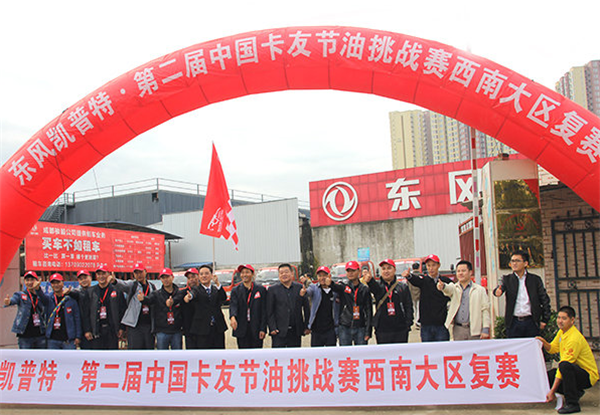活动当天，选手们早已按捺不住挑战的激情，早早来到比赛场地-成都骏毅4S店。来到比赛现场，节油一哥们认真对比赛车辆进行检查、熟悉，经过现场称油、加油、规则讲解等紧张有序的赛前准备工作后，大家都有点迫不及待、跃跃欲试。短暂而隆重的开幕式之后，在东风汽车股份有限公司党委书记陈彬的一声令下，节油一哥们鱼贯而出，开赴赛场。经过激烈的比赛，最终比赛结果出炉，最后的悬念也保持到最后一分钟。最后返回赛场的陈蓝师傅以百公里5.19L的优异成绩获得了冠军。来自重庆的曾俊和成都的曾朝义分别以百公里5.619L和百公里5.833L的成绩获得了亚军和季军，也获得参加总决赛的名额。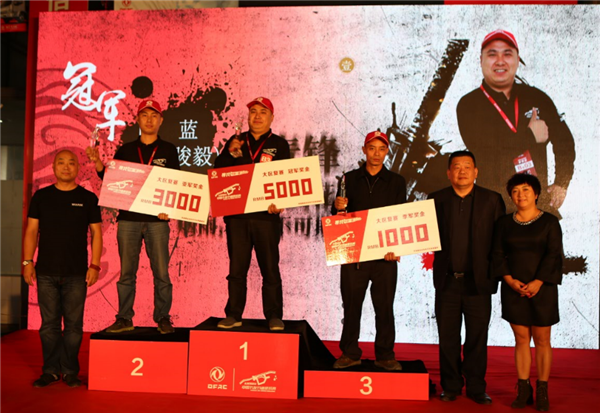西南大区复赛与前几场复赛有哪些不同呢，我们一起走进赛场，看看这些精彩亮点。

身为一个卡车司机，多数是没有节假日的，陪在妻儿身边的时间也是少之又少。节油赛既是比赛，更是卡友难得的假期，此次主办方特地邀请来节油一哥们的妻子和萌娃来到现场，她们的到来，让节油比赛充满了温情和暖意，像是一场卡友家庭欢聚的暖趴；更能让卡嫂和萌娃们了解到他们的丈夫作为轻卡司机辛苦之余，也能有除了工作以外的乐趣。当然，有了萌娃的呐喊助威，粑粑们的表现也能更精彩。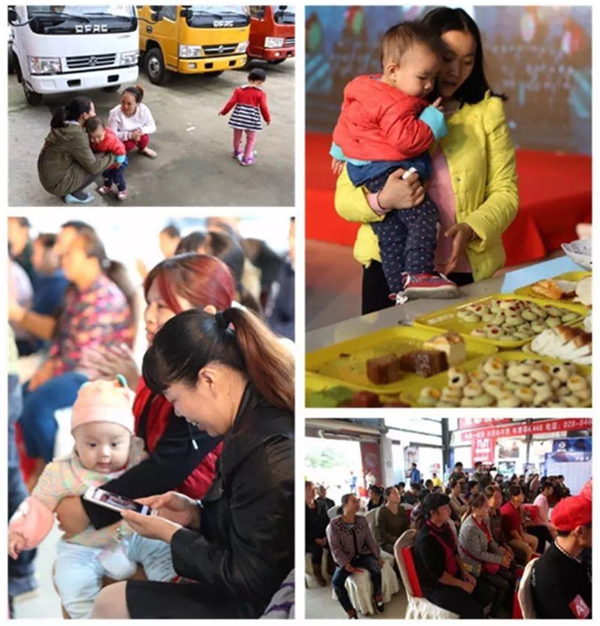比赛只是节油赛的一个环节，作为一个卡友全家聚会的“大爬梯”，当然少不了放松身心的娱乐环节。比赛之余，主办方还贴心准备了各种趣味小游戏，如微信打印照片、扔飞镖、抢椅子等，卡友们使出浑身解数，玩得热闹玩得开心，更重要的是礼品也拿到手软。温馨、开怀的互动环节让成都骏毅的大厅里面笑声不断。通过放松的互动游戏，来自各地的节油一哥们都熟悉起来，结下了非常深厚的友谊，大家互加微信互留电话，相约在以后的工作和生活中互帮互助，场面温馨而融洽。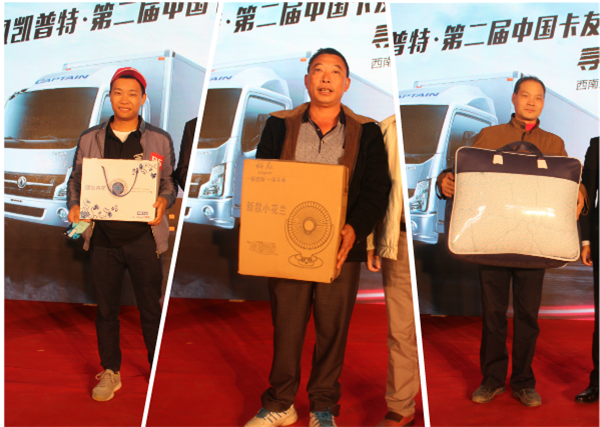特别值得一提的是，西南大区入围复赛的12位卡友中，有2位是“老伙计”了，他们就是来自成都本土的曾俊和重庆分赛区的冯道明师傅，他们曾在2015年东风汽车首届节油赛中突围而出，双双闯入总决赛。此届复赛，曾俊当仁不让，以百公里5.619L的出色节油战绩再次入围全国总决赛，而冯道明师傅以0.1L的成绩之差不敌季军得主，位列第四未能闯入复赛，令人扼腕叹息。不过冯道明师傅积极挑战节油极限的精神，为节能环保献力拼搏的赤子之心不言而喻。他与落选的其他节油一哥皆是不折不扣的节油英雄，同时也获得主办方颁发的节油优胜奖。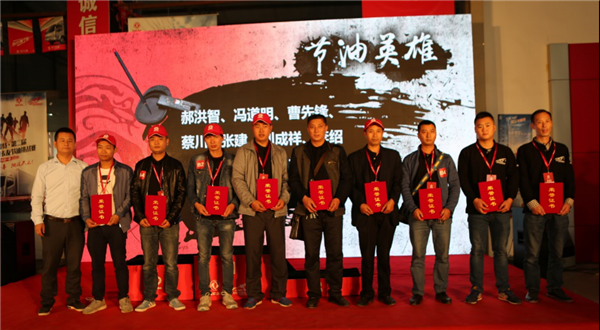俗话说，好马还得配好鞍。东风凯普特作为复赛比赛用车，已经在前四场复赛证明了其不俗节油实力。众所周知，西南赛区各分赛区多数为山区路段，卡友们日常驾驶中面临的比其他赛区更为复杂的路况，也练就了一身驾驶好本领。本届复赛，西南赛区的选手也面临着几场复赛以来最复杂的路况，有坡路段还是不少红绿灯，大家沉着冷静，三甲选手更是赛出来百公里低于6的出色战绩。好成绩也让节油一哥们对东风凯普特的节油性能交口称赞、非常认可。相信随着国V排放标准的实施，东风凯普特优异的节油性能将会成为更多轻卡司机的选择。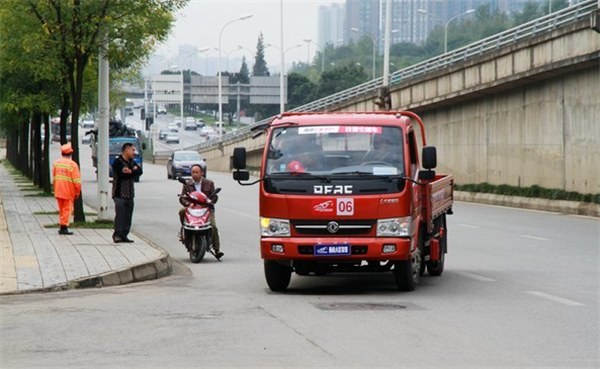每一场节油复赛，都有自己独一无二的看点，有的不断刷新节油记录，有的赛场兄弟情深，而西南大区复赛，因卡嫂和萌娃的到来又多了一份温情。这就是东风凯普特·第二届中国卡友节油挑战赛，除了节油，我们更多的是看到属于卡友们的精彩生活片段。下一场华东大区复赛将在苏州进行，在物流发达的经济重镇，又会有怎样的看点呢？让我们翘首以盼！

##### 相关资讯

|分享

•已阅！握爪
•我手滑为你点赞
•128个赞！
•不明觉厉
•阅后既醉
•有钱！任性
•照片太美，我不敢看
•干货！杠杠的
•高大上
•膜拜中！
•请接受我的膝盖
•猫哥/猫妹么么哒
•神吐槽
•我只笑笑不说话
•我想静静
•窒息
##### 资讯相关车系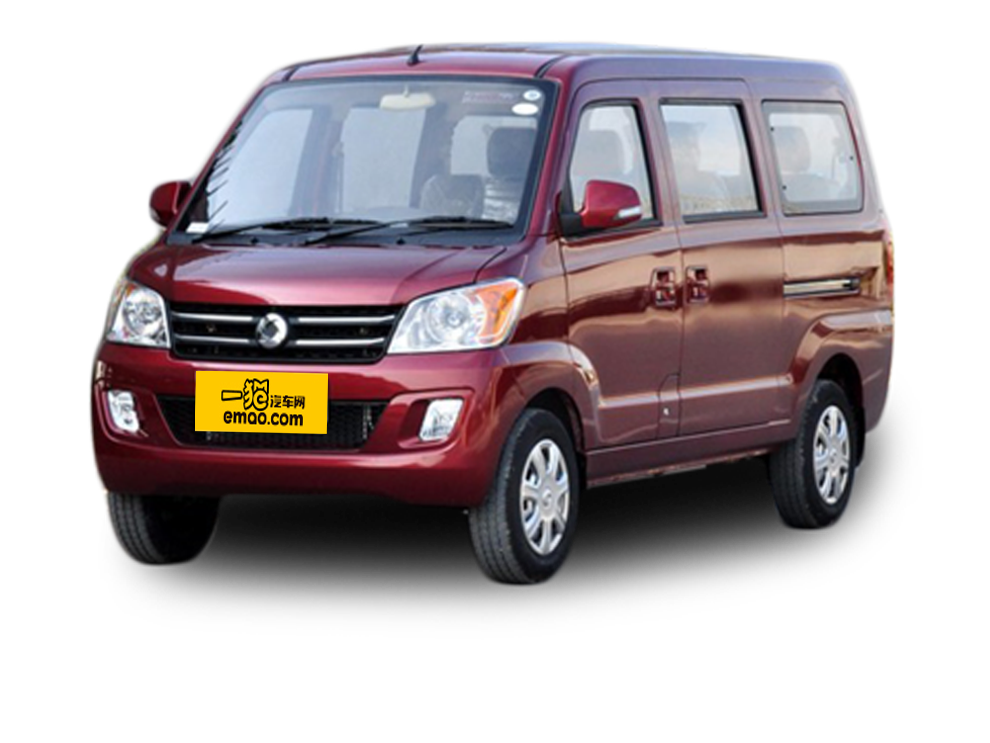##### 热度排行• 阿斯顿·马丁
• 奥迪
• 阿尔法罗密欧
• ALPINA

• 巴博斯
• 宝骏
• 宝马
• 保时捷
• 北汽制造
• 奔驰
• 奔腾
• 本田
• 比亚迪
• 标致
• 别克
• 宾利
• 布加迪
• 北汽威旺
• 北京
• 北汽绅宝
• 北汽幻速
• 北汽新能源
• 宝沃
• 比速汽车
• 北汽道达

• 昌河
• 长安
• 长城
• 长安商用
• 成功汽车
• 长江EV
• 长安轻车型

• 大众
• 道奇
• 东风
• 东风风神
• 东风小康
• 东南
• DS
• 东风风行
• 东风风度
• 东风风光

• 法拉利
• 菲亚特
• 丰田
• 福迪
• 福特
• 福田汽车
• 福汽启腾
• 风诺

• GMC
• 广汽传祺
• 广汽吉奥
• 观致

• 哈飞
• 海格
• 海马
• 华泰
• 黄海
• 恒天
• 红旗
• 哈弗
• 华颂
• 华凯
• 华泰新能源
• 汉腾汽车

• Jeep
• 江淮
• 江铃
• 捷豹
• 金杯
• 九龙
• 吉利汽车
• 金旅
• 金龙
• 江铃集团轻汽
• 江铃集团新能源
• 君马汽车
• 捷途

• 开瑞
• 凯迪拉克
• 科尼赛克
• 克莱斯勒
• KTM
• 卡威
• 凯翼
• 康迪
• 康迪电动汽车集团

• 猎豹汽车
• 兰博基尼
• 劳斯莱斯
• 雷克萨斯
• 雷诺
• 理念
• 力帆
• 莲花汽车
• 林肯
• 铃木
• 陆风
• 路虎
• 路特斯
• 领志
• 领克
• 零跑汽车

• MG
• MINI
• 马自达
• 玛莎拉蒂
• 迈凯伦
• 摩根

• 纳智捷

• 讴歌
• 欧宝
• 欧朗
• 欧拉
• 欧尚汽车

• 帕加尼

• 奇瑞
• 启辰
• 起亚
• 前途
• 庆铃汽车

• 日产
• 荣威
• 瑞麒汽车
• 如虎
• 瑞驰

• 上汽大通
• smart
• 三菱
• 双环
• 双龙
• 斯巴鲁
• 斯柯达
• 萨博
• 思铭
• 赛麟
• SWM斯威汽车

• TESLA
• 泰卡特
• 腾势

• 威麟
• 威兹曼
• 沃尔沃
• 五菱汽车
• 五十铃
• 潍柴英致
• WEY
• 蔚来
• 威马汽车

• 现代
• 雪佛兰
• 雪铁龙
• 西雅特
• 新特汽车
• 小鹏汽车
• 新宝骏

• 野马汽车
• 一汽
• 依维柯
• 英菲尼迪
• 永源
• 驭胜

• 中华
• 中兴
• 众泰
• 知豆
• 之诺
• 正道汽车
• A
• B
• C
• D
• E
• F
• G
• H
• I
• J
• K
• L
• M
• N
• O
• P
• Q
• R
• S
• T
• U
• V
• W
• X
• Y
• Z

• 阿斯顿·马丁
• 奥迪
• 阿尔法罗密欧
• ALPINA

• 巴博斯
• 宝骏
• 宝马
• 保时捷
• 北汽制造
• 奔驰
• 奔腾
• 本田
• 比亚迪
• 标致
• 别克
• 宾利
• 布加迪
• 北汽威旺
• 北京
• 北汽绅宝
• 北汽幻速
• 北汽新能源
• 宝沃
• 比速汽车
• 北汽道达

• 昌河
• 长安
• 长城
• 长安商用
• 成功汽车
• 长江EV
• 长安轻车型

• 大众
• 道奇
• 东风
• 东风风神
• 东风小康
• 东南
• DS
• 东风风行
• 东风风度
• 东风风光

• 法拉利
• 菲亚特
• 丰田
• 福迪
• 福特
• 福田汽车
• 福汽启腾
• 风诺

• GMC
• 广汽传祺
• 广汽吉奥
• 观致

• 哈飞
• 海格
• 海马
• 华泰
• 黄海
• 恒天
• 红旗
• 哈弗
• 华颂
• 华凯
• 华泰新能源
• 汉腾汽车

• Jeep
• 江淮
• 江铃
• 捷豹
• 金杯
• 九龙
• 吉利汽车
• 金旅
• 金龙
• 江铃集团轻汽
• 江铃集团新能源
• 君马汽车
• 捷途

• 开瑞
• 凯迪拉克
• 科尼赛克
• 克莱斯勒
• KTM
• 卡威
• 凯翼
• 康迪
• 康迪电动汽车集团

• 猎豹汽车
• 兰博基尼
• 劳斯莱斯
• 雷克萨斯
• 雷诺
• 理念
• 力帆
• 莲花汽车
• 林肯
• 铃木
• 陆风
• 路虎
• 路特斯
• 领志
• 领克
• 零跑汽车

• MG
• MINI
• 马自达
• 玛莎拉蒂
• 迈凯伦
• 摩根

• 纳智捷

• 讴歌
• 欧宝
• 欧朗
• 欧拉
• 欧尚汽车

• 帕加尼

• 奇瑞
• 启辰
• 起亚
• 前途
• 庆铃汽车

• 日产
• 荣威
• 瑞麒汽车
• 如虎
• 瑞驰

• 上汽大通
• smart
• 三菱
• 双环
• 双龙
• 斯巴鲁
• 斯柯达
• 萨博
• 思铭
• 赛麟
• SWM斯威汽车

• TESLA
• 泰卡特
• 腾势

• 威麟
• 威兹曼
• 沃尔沃
• 五菱汽车
• 五十铃
• 潍柴英致
• WEY
• 蔚来
• 威马汽车

• 现代
• 雪佛兰
• 雪铁龙
• 西雅特
• 新特汽车
• 小鹏汽车
• 新宝骏

• 野马汽车
• 一汽
• 依维柯
• 英菲尼迪
• 永源
• 驭胜

• 中华
• 中兴
• 众泰
• 知豆
• 之诺
• 正道汽车
• A
• B
• C
• D
• E
• F
• G
• H
• I
• J
• K
• L
• M
• N
• O
• P
• Q
• R
• S
• T
• U
• V
• W
• X
• Y
• Z

• 阿斯顿·马丁
• 奥迪
• 阿尔法罗密欧
• ALPINA

• 巴博斯
• 宝骏
• 宝马
• 保时捷
• 北汽制造
• 奔驰
• 奔腾
• 本田
• 比亚迪
• 标致
• 别克
• 宾利
• 布加迪
• 北汽威旺
• 北京
• 北汽绅宝
• 北汽幻速
• 北汽新能源
• 宝沃
• 比速汽车
• 北汽道达

• 昌河
• 长安
• 长城
• 长安商用
• 成功汽车
• 长江EV
• 长安轻车型

• 大众
• 道奇
• 东风
• 东风风神
• 东风小康
• 东南
• DS
• 东风风行
• 东风风度
• 东风风光

• 法拉利
• 菲亚特
• 丰田
• 福迪
• 福特
• 福田汽车
• 福汽启腾
• 风诺

• GMC
• 广汽传祺
• 广汽吉奥
• 观致

• 哈飞
• 海格
• 海马
• 华泰
• 黄海
• 恒天
• 红旗
• 哈弗
• 华颂
• 华凯
• 华泰新能源
• 汉腾汽车

• Jeep
• 江淮
• 江铃
• 捷豹
• 金杯
• 九龙
• 吉利汽车
• 金旅
• 金龙
• 江铃集团轻汽
• 江铃集团新能源
• 君马汽车
• 捷途

• 开瑞
• 凯迪拉克
• 科尼赛克
• 克莱斯勒
• KTM
• 卡威
• 凯翼
• 康迪
• 康迪电动汽车集团

• 猎豹汽车
• 兰博基尼
• 劳斯莱斯
• 雷克萨斯
• 雷诺
• 理念
• 力帆
• 莲花汽车
• 林肯
• 铃木
• 陆风
• 路虎
• 路特斯
• 领志
• 领克
• 零跑汽车

• MG
• MINI
• 马自达
• 玛莎拉蒂
• 迈凯伦
• 摩根

• 纳智捷

• 讴歌
• 欧宝
• 欧朗
• 欧拉
• 欧尚汽车

• 帕加尼

• 奇瑞
• 启辰
• 起亚
• 前途
• 庆铃汽车

• 日产
• 荣威
• 瑞麒汽车
• 如虎
• 瑞驰

• 上汽大通
• smart
• 三菱
• 双环
• 双龙
• 斯巴鲁
• 斯柯达
• 萨博
• 思铭
• 赛麟
• SWM斯威汽车

• TESLA
• 泰卡特
• 腾势

• 威麟
• 威兹曼
• 沃尔沃
• 五菱汽车
• 五十铃
• 潍柴英致
• WEY
• 蔚来
• 威马汽车

• 现代
• 雪佛兰
• 雪铁龙
• 西雅特
• 新特汽车
• 小鹏汽车
• 新宝骏

• 野马汽车
• 一汽
• 依维柯
• 英菲尼迪
• 永源
• 驭胜

• 中华
• 中兴
• 众泰
• 知豆
• 之诺
• 正道汽车
• A
• B
• C
• D
• E
• F
• G
• H
• I
• J
• K
• L
• M
• N
• O
• P
• Q
• R
• S
• T
• U
• V
• W
• X
• Y
• Z